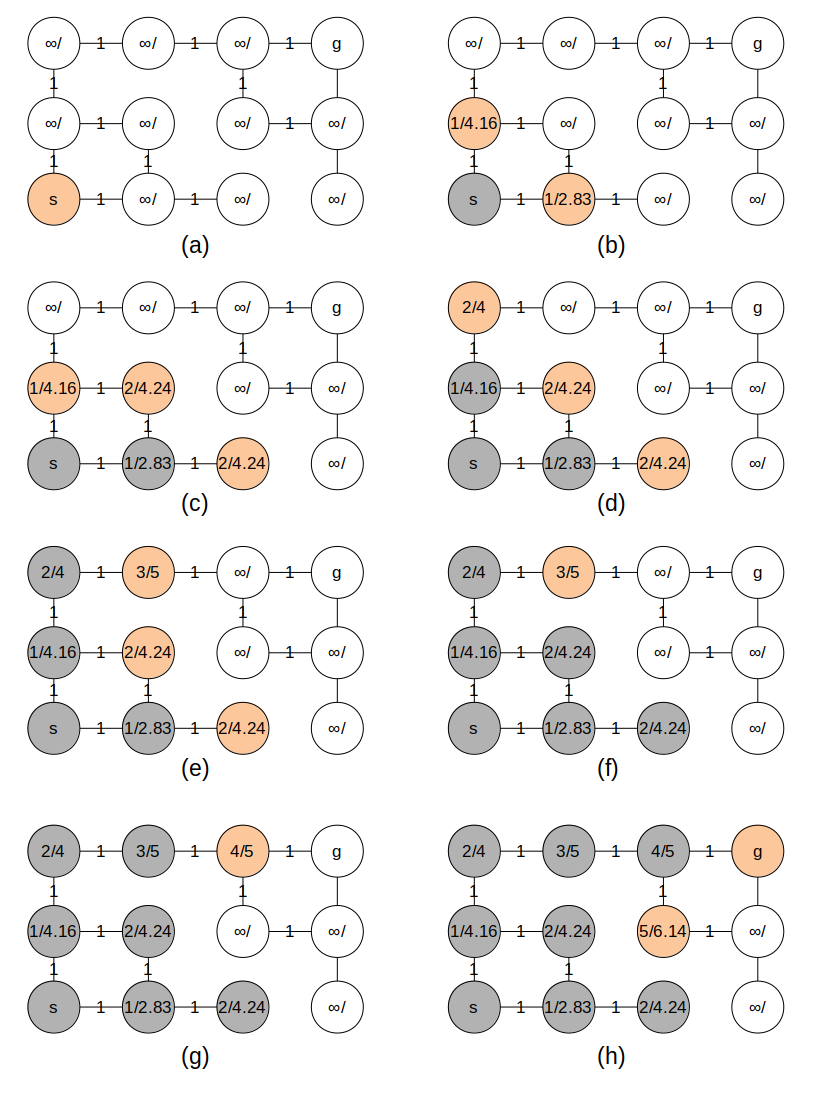# 详解寻路算法(1)-图搜索

## 1. 引言

1. 地图 抽象成 . 这里的图指的是数学上的图 $G=(V,E)$;
2. 对图进行搜索.

## 2. Dijkstra 算法

### 2.1 松弛操作

Dijkstra 和 A* 算法都要用到松弛操作. 对于每个节点 v, 我们维持一个属性 v.d, 用于记录从源节点 s 到节点 v 的最短路径的长度上界(意思是最短路径有多长我们暂时不知道;但是肯定小于这个值). 我们称其为最短路径估计. 初始时, 原点的 最短路径估计 被赋为 0, 其余的都被赋为无穷大. 除此之外, 每个节点都有一个 parent 属性, v.parant 表示最短路径中 v 的前序. 初始时 v.parent 赋值为 None.

def dijk_init(graph, s):
for v in graph.vertices:
v.d = float('inf')
v.parent = None
s.d = 0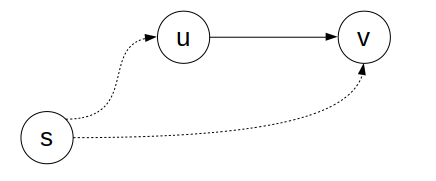new_d = u.d + graph.w(u, v)
if new_d < v.d:
v.d = new_d
v.parent = u


### 2.2 贪心策略

Dijkstra 算法是一个典型的贪心算法. 它总是维护一个优先队列. 初始时, 这个队列中只有源节点; 之后不断地从队列中取出最短路径估计最小的节点, 松弛其所有的临边, 如果某个节点 v 在松弛操作中改变了 v.d, 就把他加入队列, 直到队列为空. 代码如下:

def dijk(graph, s):
dijk_init(graph, s)
queue = PriorityQueue()
queue.put(s, s.d)

while not queue.empty():
u = queue.get()
new_d = u.d + graph.w(u, v)
if new_d < v.d:
v.d = new_d
v.parent = u
queue.put(v, v.d)


I eliminate the check for a node being in the frontier with a higher cost. By not checking, I end up with duplicate elements in the frontier. The algorithm still works. It will revisit some locations more than necessary (but rarely, in my experience, as long as the heuristic is admissible). The code is simpler and it allows me to use a simpler and faster priority queue that does not support the decrease-key operation. The paper "Priority Queues and Dijkstra’s Algorithm" suggests that this approach is faster in practice.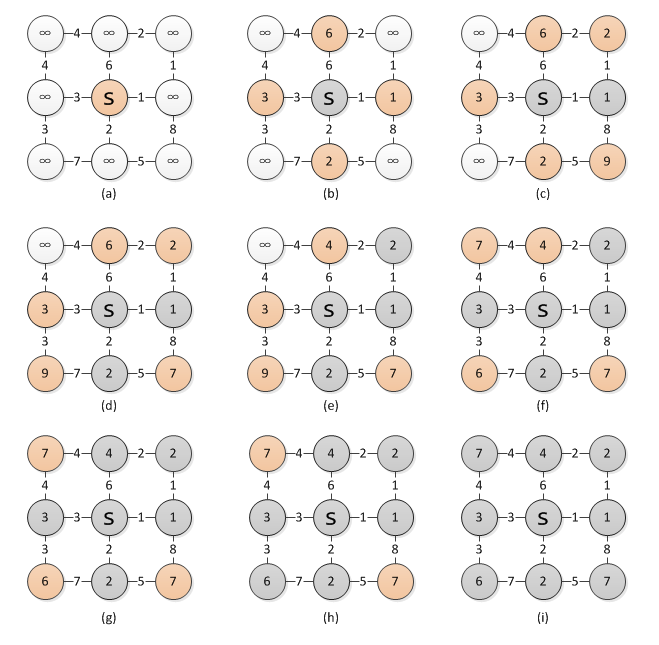## 3. A* 算法

### 3.1 启发式搜索

def heuristic(a, b):
return math.sqrt((a.x - b.x) ** 2 + (a.y - b.y) ** 2)


def greedy(graph, s, g):
greedy_init(graph, s) # set v.parent = None for all vertices
queue = PriorityQueue()
queue.put(s, 0)

while not queue.empty():
u = queue.get()
if u == g: break
if not v.parent:
h = heuristic(v, g)
v.parent = u
queue.put(v, h)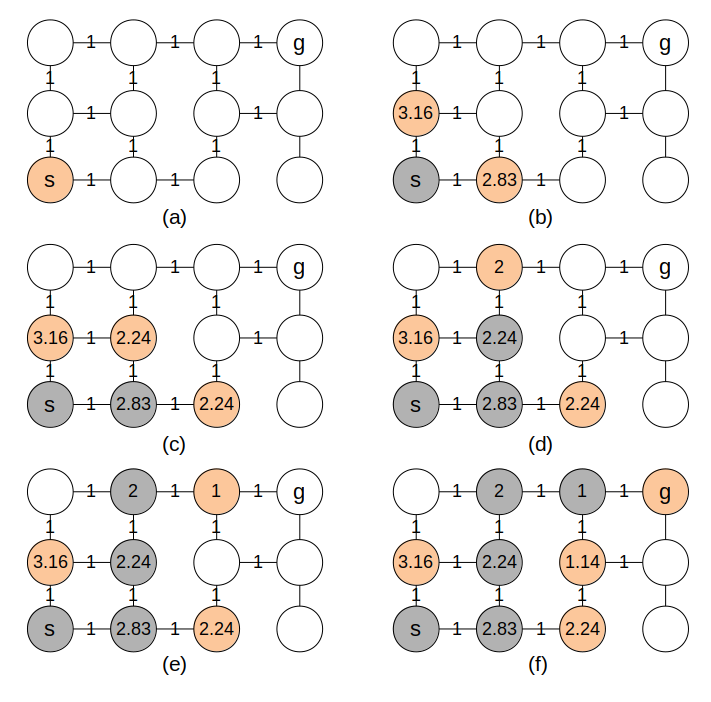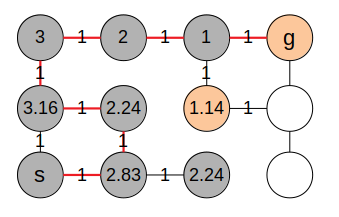### 3.2 A* 算法

Dijkstra 算法保证能找到最短路径, 但是却探索了所有的节点, 浪费了时间; 而贪婪优先搜索只朝着目标节点探索, 却不能保证结果的正确性. 把这两者相结合, 便是今天的主角 – A* 算法了. 下面是 A* 算法的代码, 你会发现它和 Dijkstra 算法的代码非常相似:

def astar(graph, s, g):
astar_init(graph, s) # as same as dijk_init
queue = PriorityQueue()
queue.put(s, s.d)

while not queue.empty():
u = queue.get()
new_d = u.d + graph.w(u, v)
if new_d < v.d:
v.d = new_d
v.parent = u
queue.put(v, v.d + heuristic(v, g))